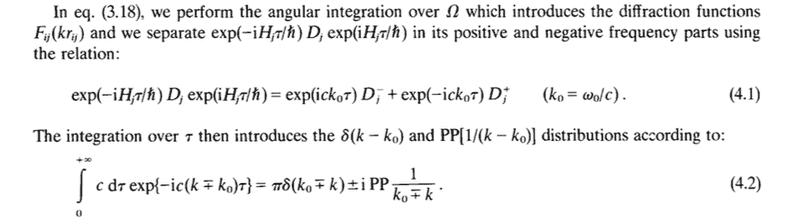# Question on derivation/notation in a reportThis is from "An Essay on the Theory of Collective Spontaneous Emission" by Gross and Haroche (1982).

I was wondering how exactly they were able to separate the operator D in the Heisenberg representation into positive and negative frequencies with an imaginary exponential on only the left side of ## D^{\pm}_j ##.

I was also wondering what exactly the PP distribution was referring to. I don't recall reading it defined earlier, and am not entirely sure what it is.

Any help with the above would be greatly appreciated.

vanhees71
Gold Member
2021 Award
Let's set ##c=1## to simplify things and then regularize the integral by introducing a small negative imaginary part to ##k## then you have the integral
$$I(k-k_0)=\int_0^{\infty} \mathrm{d} \tau \exp[-\mathrm{i} \tau (k-k_0-\mathrm{i} 0^+)]=\frac{1}{\mathrm{i}(k-k_0+\mathrm{i} 0^+)}=-\pi \delta(k-k_0) - \mathrm{i} \text{PP} \frac{1}{k-k_0}.$$

Let's set ##c=1## to simplify things and then regularize the integral by introducing a small negative imaginary part to ##k## then you have the integral
$$I(k-k_0)=\int_0^{\infty} \mathrm{d} \tau \exp[-\mathrm{i} \tau (k-k_0-\mathrm{i} 0^+)]=\frac{1}{\mathrm{i}(k-k_0+\mathrm{i} 0^+)}=-\pi \delta(k-k_0) - \mathrm{i} \text{PP} \frac{1}{k-k_0}.$$

Thank you for the response. I think this is fairly basic, but how exactly did you evaluate

$$\frac{e^{-\mathrm{i} \tau (k-k_0-\mathrm{i} 0^+)}}{-\mathrm{i}(k-k_0 - \mathrm{i} 0^+)}$$

in the limit ## \tau \rightarrow \infty ##?

And I think this is just stemming from my lack of understanding, but how exactly are the two expressions: ##\frac{1}{\mathrm{i}(k-k_0+\mathrm{i} 0^+)}## and ## -\pi \delta(k-k_0) - \mathrm{i} \text{PP} \frac{1}{k-k_0} ## equivalent?

George Jones
Staff Emeritus
Gold Member
•vanhees71
Gold Member
2021 Award
Thank you for the response. I think this is fairly basic, but how exactly did you evaluate

$$\frac{e^{-\mathrm{i} \tau (k-k_0-\mathrm{i} 0^+)}}{-\mathrm{i}(k-k_0 - \mathrm{i} 0^+)}$$

in the limit ## \tau \rightarrow \infty ##?

And I think this is just stemming from my lack of understanding, but how exactly are the two expressions: ##\frac{1}{\mathrm{i}(k-k_0+\mathrm{i} 0^+)}## and ## -\pi \delta(k-k_0) - \mathrm{i} \text{PP} \frac{1}{k-k_0} ## equivalent?

Concerning the 1st question: That's the point of introducing the ##-\mathrm{i} 0^+##. No matter how small you take it, it makes the exponential vanish in the limit ##\tau \rightarrow \infty##. That's how you get the final result as the integral only taken at the lower limit ##\tau=0##.

Concerning the 2nd question: Take an analytic test function ##\phi(k)## vanishing quickly enough for (complex!) ##k## anywere. Now we want to evaluate the integral
$$\Phi_{\epsilon}(k_0)=\int_{-\infty}^{\infty} \mathrm{d} k \frac{f(k)}{k-k_0+\mathrm{i} \epsilon}.$$
Now we have
$$\frac{1}{k-k_0+\mathrm{i} \epsilon}=\frac{k-k_0-\mathrm{i} \epsilon}{(k-k_0)^2+\epsilon^2}.$$
Thus we can write the integral as
$$\Phi_{\epsilon}(k_0)=\int_{-\infty}^{\infty} \mathrm{d} k \left [\frac{f(k)}{k-k_0} \; \frac{(k-k_0)^2}{(k-k_0)^2+\epsilon^2}-\frac{\mathrm{i} \epsilon}{(k-k_0)^2+\epsilon^2} f(k) \right].$$
For the first term the contributions from ##|k-k_0| \gg \epsilon## the 2nd factor is ##\approx 1## while for ##|k-k_0|^2 \ll \epsilon## the contribution is negligible. For ##\epsilon \rightarrow 0^+## you get thus from this term the principle value, because the 2nd factor is symmetric around ##k=k_0##.

In the 2nd term the factor in the integrand is an approximate ##\delta## function, since
$$\int_{-\infty}^{\infty} \mathrm{d} k \frac{\epsilon}{(k-k_0)^2+\epsilon^2}=\arctan \left (\frac{k-k_0}{\epsilon} \right)_{-\infty}^{\infty}=\pi,$$
and for ##\epsilon \rightarrow 0^+## this factor goes to 0 for ##k-k_0 \neq 0## and to ##\infty## for ##k-k_0=0##. So finally we have
$$\lim_{\epsilon \rightarrow 0^+} \int_{-\infty}^{\infty} \mathrm{d} k \frac{f(k)}{k-k_0+\mathrm{i} \epsilon} = \text{PP} \int_{-\infty}^{\infty} \mathrm{d} k \frac{f(k)}{k-k_0} -\mathrm{i} \pi f(k_0),$$
and this we wanted to show.

Concerning the 1st question: That's the point of introducing the −i0+−i0+-\mathrm{i} 0^+. No matter how small you take it, it makes the exponential vanish in the limit τ→∞τ→∞\tau \rightarrow \infty. That's how you get the final result as the integral only taken at the lower limit τ=0τ=0\tau=0.

Thank you for the explanation. That was incredibly helpful! I think I'm just missing this initial part: how is it valid to introduce ##\mathrm{i} 0^+## into the expression? If both both limits ##\tau \rightarrow \infty ## and ##0^+ \rightarrow 0## are necessary to evaluate the original integral, we just have to ensure ##\tau## is increasing more quickly than ##0^+## is decreasing in order to be able to evaluate the integral only at ##\tau = 0##, correct? Regularization of the integral is not something I am too familiar with, but thank you for sharing.

vanhees71
•